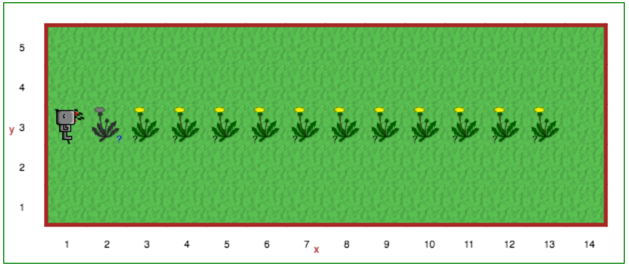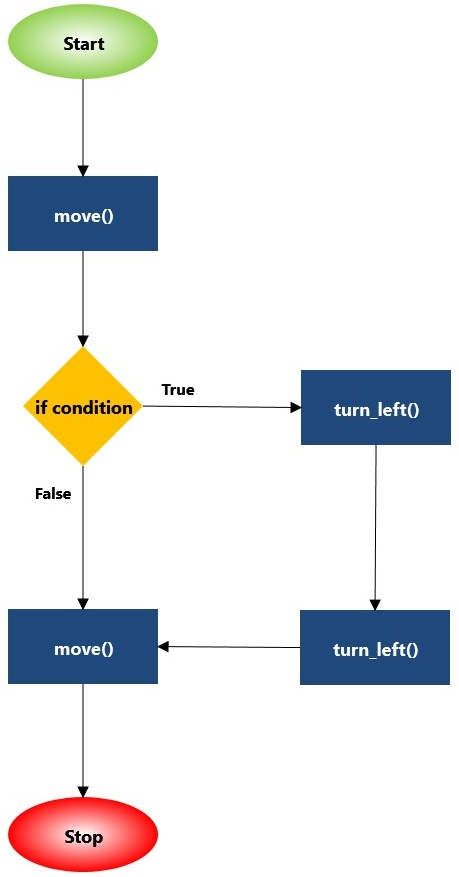## Tutorial¶

Sometimes, we don’t know ahead of time exactly what the world that Reeborg is going to be faced with will look like. Thankfully, Reeborg can sense the world around it, and we can ask Reeborg questions about it. To ask the computer a question, we use an `if` statement. The so-called `if` statement follows a pattern somewhat similar to that of `function`s :

```def some_name():
# block of code

if some_condition:
# block of code
```

Note

The general term used to describe a function that gives a result equivalent to `True` or `False` in an `if` statement is condition:

```if condition:
...
```

Having to specify `True` or `False` does not help Reeborg decide on its own. However, there are special functions that Reeborg recognizes that allow Reeborg to make decisions. The first of these is `object_here()` which tells Reeborg if there is at least one object at the grid position where it is located. For example, if we want to ask Reeborg to collect objects (dandelions, carrots, etc), one part of the code could be:

```if object_here():
take()
```

The complete list of functions (questions) that Reeborg can answer is as follows:

Question/Function

Explanation

at_goal()

Returns True if Reeborg is on a goal tile (flag, home, or green tile), False otherwise.

front_is_clear()

Returns True if there is no obstacle (wall, etc) in front of Reeborg, False otherwise.

right_is_clear()

Returns True if there is no obstacle (wall, etc) to the right of Reeborg, False otherwise.

wall_in_front()

Returns True if there is a wall in front of Reeborg, False otherwise.

wall_on_right()

Returns True if there is a wall on the right side of Reeborg, False otherwise.

object_here()

Returns True if there is an object (star, dandelion, etc) on the same tile as Reeborg is standing on, False otherwise.

carries_object()

Returns True if Reeborg is currently carrying at least one object (star, dandelion, etc). Returns False if Reeborg is carrying nothing.

is_facing_north()

Returns True if Reeborg is currently facing north, False otherwise.

Open Step 8 on the Reeborg environment.Reeborg’s friend Bree loves to pick dandelions and blow the seeds as far as she can. Because of this, Reeborg often finds dandelions growing in the front yard. The dandelions aren’t always in the same spot, though. To see this, press the play button on the Step 8 world, and notice that dandelions appear in different places each time. You can tell that this is going to happen because of the black question mark underneath the image of the dandelion.

Create a program to have Reeborg walk across the front yard, picking up a dandelion whenever it finds one. There might be a dandelion in any of the locations that have a dandelion image with a question mark underneath (you can see this again if you press the reload button). You will need to ask Reeborg questions as it moves across the front yard. Once every possible dandelion is picked up, have Reeborg put down all the dandelions it has picked up at (2,3), then return to the flag (1,3). Remember to use comments and whitespace to increase the readability of your solution!

## If You’re Having Trouble (a more detailed explanation)¶

```if True:
move()

if False:
turn_left()
```

In the code above, `if`, `True`, and `False` are all Python keywords. It is a good idea to try out the code above in Reeborg’s World (you can use any world you like, perhaps Alone?). You might also want to interchange the True and False, then run the program again to see what happens.

When we introduced functions, we explained how we could think of a function call as being somewhat equivalent to inserting the code block for the function definition at that point in the program. Thus:

```move()
turn_right()  # function call
move()
```

is equivalent to:

```move()
# begin of code block inside turn_right()
turn_left()
turn_left()
turn_left()
# end of code block
move()
```

`if` statements can be thought in similar terms, except that we have a conditional insertion (or rather deletion!). Thus:

```move()
if True:
turn_left()
turn_left()
move()
```

is equivalent to:

```move()
turn_left()
turn_left()
move()
```

whereas:

```move()
if False:
turn_left()
turn_left()
move()
```

is equivalent to:

```move()
move()
```

Note that thinking of it this way does not mean that such a deletion would be done permanently: if, somehow, our program looped back and repeated this part of the code again, the `if` statement would be reevaluated each time to decide whether or not to execute the lines of code inside the code block.

We can represent the above using a flowchart:Next Section - Step 9: Repeating Instructions